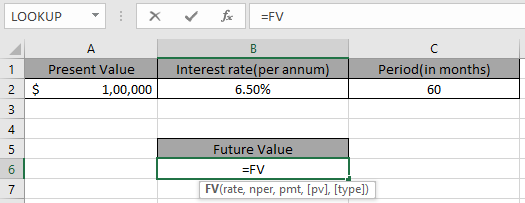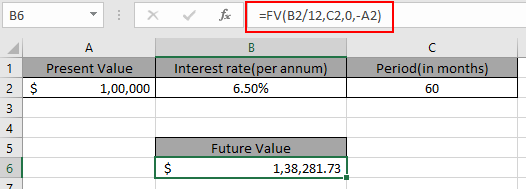# How to Use FV function in Excel

In this article, we will learn about how to find the future value of the amount using FV function in Excel.

Future value function returns the future value of the present amount having interest rate over a period.
Syntax:

=FV (rate, nper, pmt, [pv], [type])

rate: Interest rate per period
nper: total no. of payment period.
pmt: amount paid each period.
pv - [optional] The present value of future payments must be entered as a negative number.
type - [optional] When any amount is due at the end of the payment. Default is 0.
Use this in an example to understand this.

Here we have a Present value amount, Interest rate and period on which we need to calculate future value of the amountUse the formula in B6 cell.

=FV(B2/12,C2,0,-A2)

Explanation:
B2/12: rate is divided by 12 as we are calculating interest for monthly periods.
-A2: principal amount is in negative so as to get the future value in positive.

And press EnterFuture value of \$100,000 at an Interest rate of 6.5% in 5 years will be \$138,281.73
Hope you understood How to use FV function excel to get the future value of the data. Explore more articles here on Excel financial. Please feel free to write your queries in the comment box below. We will help you.

Related Articles:

How to Use PV function in Excel

How to use XNPV function in Excel

How to use the Excel IPMT Function

How to Use Compound Interest Function in Excel

Popular Articles:

How to use the VLOOKUP Function in Excel

How to use the COUNTIF function in Excel 2016

How to Use SUMIF Function in Excel

Terms and Conditions of use

The applications/code on this site are distributed as is and without warranties or liability. In no event shall the owner of the copyrights, or the authors of the applications/code be liable for any loss of profit, any problems or any damage resulting from the use or evaluation of the applications/code.